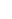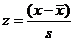# Day 12

GOB: Watch decathlon footage from the 2008 Summer Olympic games.   /* I spot check A12 */

Who is taller  ____________________ or _____________________ ?

-------------------------------------------------------------

Strategies for gold, silver, bronze:

We have always been told that you can’t compare ___________________ to __________________

But in AP Statistics __________________.

 Adding (subtracting) a constant to every data value __________ the same constant to measures of position/center and _________ measures of spread. Javelin adds (subtracts)                                              Translation (Shift) does not change Multiplying (dividing) every data value by a constant __________ the same constant to measures of position/center and __________ measures of spread. High Jump   multiplies (divides)                                               Dilation multiplies (divides) Changing the center and spread of a variable is equivalent to __ changing its units.Lets go back to the 100m results of the decathlon (hopefully it is still saved in a list).  Redraw the histogram to the right using the following window values:  Xmin=10, Xmax=12.25, Xscl=.25, Ymin= -2, Ymax=16, Yscl=1, Xres=1

Draw an arrow to label the location of each athlete’s result.

It would be nice if we could find a measure that would tell us how unusual a result is

and one that would enable us to compare results across different events.How unusual a result is depends on its deviation, distance from the mean.  We can create a new histogram using x –  to shift the data so the (center) mean = 0, but it still won’t let us compare results from different events because the (spreads) standard deviations will still be different.  To fix that problem we have to divide the data by the standard deviation as well.

TI 83/84:  (L1 – 11.118)/0.329367, STO →, 2nd, L2, ENTER    (the new data is now in L2!)

TI 89:  (VAR-LINK), (select list1 – 11.118)/0.329367, STO →, 2nd, - (VAR-LINK), select list2, ENTER (new data in L2!)

Now make histogram of L2 to the right using the following window values: Xmin=-3.6, Xmax=3.6, Xscl=.8,

Ymin=-2, Ymax=16, Yscl=1, Xres=1

 Standardizing Uses the standard deviation as a ruler to measure distance from the mean creating z-scoresz-scores tell us _____________ important uses are: the number of standard deviations a value is from the mean. 1. Comparing values from different distributions (decathlon events)     or values based on different units. 2. Identifying unusual or surprising values among data. 3. Converting standard deviations into percentiles with the     Standard normal (Distribution) probabilities table. Units can be eliminated by ____ __________ have no units. standardizing the data. z-scores When we standardize data to get  _____ we do two things.  First we _____ the data by subtracting the mean.  Then we _____ the data by dividing by their standard deviation. z-scores shift   rescale Standardizing has the following affect on the distribution of a variable: Shape – is not changed. Center – the mean is shifted to 0 Spread – the standard deviation is rescaled to 1

# A12

1) Complete all the problems on the class handout.

1) Use the original, Day 12, table of results for the decathlon to write in the z-score for each athlete below.

 100 Metres High Jump Javelin Total Leonel Suarez Bryan Clay Andrei Krauchanka

For example, to calculate Leonel Suarez’s

z-score for the 100 Metres:

Who would be the winner if these were the only three events?  Why?

Also who turned in the most remarkable performance of these three events? How did you determine it?

2) The heights of 18year-old men are unimodal and approximately symmetric with mean 68 inches and standard deviation 3 inches.  The heights of 18year-old women are unimodal and approximately symmetric with mean 66.7 inches and standard deviation 3.2 inches.        ___________ is ______ tall and ___________ is ______ tall, who is tallest?